## 2010年11月19日 星期五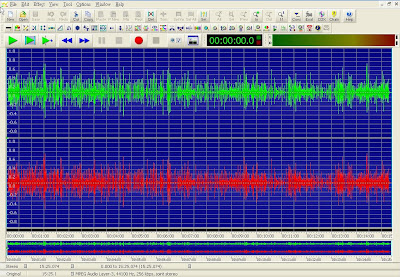1. 使用"選擇區段設定"按鍵，進行輸入選擇區段如下圖2. 使用滑鼠右鍵選擇開始標記和結束標記，標示選擇區段
3. 在聲音圖上按下滑鼠左鍵不放，然後左移至選擇區段之最左端，再右移至選擇區段之最右端如下圖， 最後放掉滑鼠左鍵，即可選擇。
選擇完成後如下圖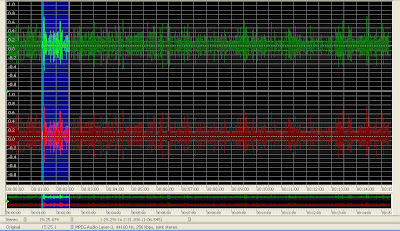上圖中包含兩大區塊：上區塊為觀看區，下區塊為目前訊號全部狀態。
選擇完成後可以"觀看選擇區段"或"放大"按鍵再次觀看所選擇區段是否正確，若需修正可以滑鼠左鍵拉拖選擇區段之左端與右端。至於正確與否，可用"播放全部聲音訊號"和"播放選擇區段聲音訊號"按鍵聽取並搜尋，記下如前圖中播放時間軸的時間位置，再修正選擇區段及完成了區段選定。最後完成如下圖上圖中之上區塊為全部觀看區段，藍色部分為選擇區段，黑色為未被選取區段，下區塊為全部訊號，而白色框線為觀看區段的標示區，可以滑鼠左鍵拉拖改變觀看區段大小。上下區塊皆可以滑鼠左鍵拉拖改變選擇區段大小。
最後再將選擇區段以"選擇區段刪除"或"選擇區段保留"按鍵刪除或保留、其餘刪除，如下圖刪除選擇區段。再進行存檔動作 即可。## 2010年11月14日 星期日

### density factor...

>>fdatool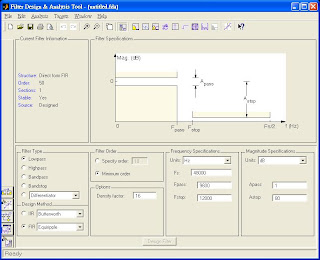Matlab的說明指出：The FIR Equiripple filter has a Density Factor option which controls the density of the frequency grid. Increasing the value creates a filter which more closely approximates an ideal equiripple filter, but more time is required as the computation increases.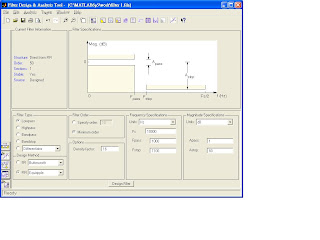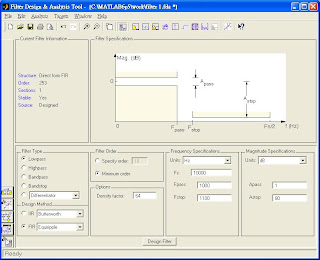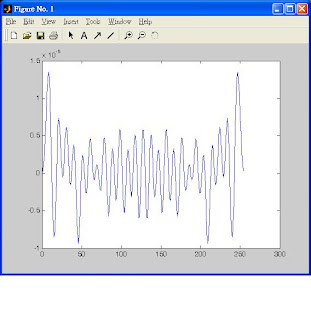## 2010年4月28日 星期三% collision simulation
clear;

for kk=1:100;
for t=1:100;
x=zeros(60,1);
y=zeros(60,1);
% 由60個中隨機抽5個
for j=1:5;
flg=1;
while flg==1;
ix(j)=ceil(rand(1,1)*60); %抽一個數字
if x(ix)==1;
flg=1;
else
flg=0;
x(ix(j))=1;
end;
end

end

for j=1:5;
flg=1;
while flg==1;
iy(j)=ceil(rand(1,1)*60); %抽一個數字
if y(iy)==1;
flg=1;
else
flg=0;
y(iy(j))=1;
end;
end

end

count=0;
for jj=1:60;
if x(jj)==1 & y(jj)==1,
count=count+1;
end
end
z(t)=count;

end
% histogram
count1=zeros(1,6);
for iz=1:100;
switch z(iz);
case 0;count1(1)=count1(1)+1;
case 1; count1(2)=count1(2)+1;
case 2; count1(3)=count1(3)+1;
case 3; count1(4)=count1(4)+1;
case 4; count1(5)=count1(5)+1;
case 5; count1(6)=count1(6)+1;
end
end

no_col(kk,:)=count1;

end
mean(no_col)/100

## 2010年4月27日 星期二

99.04. 27(不能打方程式?)

(2)只有一個桶子中同時有紅球和藍球的機率=遇見一次的機率